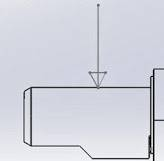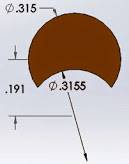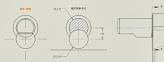# Maximum force

JGrant
Hello,

If I have a shaft that has a section area of .0562 in. sq. and a material yield strength of 60KSI.
And I will have the force applied at the top of the shaft pushing down.
The shaft will be in a cantilever configuration where the force will be applied .125" from the base of the shaft.
How to I calculate the maximum force that can be applied to the shaft before exceeding the yield strength?
Also the shaft is not a perfect circle. It is a circle with an radial under cut.

Thank you.

JGrant
I know that if I pull straight out on this shaft that the shear force would be 3370.2 LBS [60,000PSI (ultimate str) X .05617 in sq (area)].
But I am trying to calculate the max force applied on the top of the shaft.
Would that be: 3370.2LBS X .125 inches = 421.275 in-lbs? but this is in torque when Im looking for the max shear force.
If it helps I used a hydraulic press to snap one off and it took approx. 1/2 ton. The gauge can only easily read tons in 1/10 fractions. ie 200lb marks.

Any help would be greatly appreciated.
Thanks

Homework Helper
JGrant: Does your round shaft and radial undercut look like the attached diagram? Is the perpendicular applied force (P), on your cantilever shaft, located at exactly 3.175 mm from the centerline of the radial undercut, as shown in the attached diagram?

Is the round shaft diameter d1 = 6.7927 mm, as shown in the diagram? If not, what is diameter d1? What is diameter d2, shown in the attached diagram, at the trough (deepest point) of the undercut? Also, what is the undercut radius?

What did you mean by, "The shaft is not a perfect circle"? If any vertical cross section, through the attached diagram, would not be a perfect circle, then could you post a dimensioned sketch of the undercut cross section shape, showing the correct orientation of the shape relative to the applied force?

#### Attachments

•shaft-01.png
731 bytes · Views: 597
Last edited:
•1 person
JGrantNVN,

The first pic is the direction of the force. The 2nd pic is the cross section view of the shaft.
The undercut is not like in your pic. Sorry for the misunderstanding.

Yes the force is located at distance 3.175 mm (.125 in), but the diameter is 8mm (.315in) prior to the undercut, which is shown in the 2nd pic.

What I meant by the shaft isn't a perfect circle is that it is moon shaped.

I hope this info helps.

Thank you

Homework Helper
JGrant: Where did your shaft break? Did it break where I drew a pink line? Can you draw a red line where the shaft broke?

And wherever you draw the red line, then we need to see a dimensioned cross section at that location.

The brown cross section you provided looks nice. But we do not know where that section is located on your first diagram. Also, your shaft appears to have a slit, where I drew a red arrow. Therefore, we need the shape and dimensions of the shaft cross section(s), as the shaft enters the plate.

And is the shaft in contact on the plate at the slit, where I drew the red arrow? Or is there a gap there, such that the shaft is not in contact there? If in contact, we need to see the dimensioned shape where it is in contact.

#### Attachments

Last edited:
JGrantSorry for the confusion, I thought I was making it simpler to understand.
The image shows where the cross section is at and the slit is from the undercut which only goes into the shaft so far. The undercut is from a hole drilled out from the opposite side of the shaft.
The part is actually powdered metal cast, so the shaft does not go thru a plate.
The shaft snapped off right at the section line E-E. Or basically the base of the shaft.

So for this problem it is like a cantilever beam(with the shaft beaming the beam).

I hope this clears things up.

Homework Helper
JGrant: I currently obtained the following. Neutral axis location is at yb = 0.9369 mm above the upper circle centerpoint. Second moment of area, I = 79.15 mm^4. Extreme fiber distances, c1 = 3.067 mm, c2 = -3.363 mm. I think this is a brittle material (compressive strength higher than tensile strength), so I would say you can ignore c2, and only use c1, the tensile extreme fiber.

What is the tensile ultimate strength (Stu) of your material?

From your test results, we know your shaft snaps when bending stress sigma = M*c1/I = 547 MPa, which occurs when you apply a force of Pu = 4448 N.

Nonetheless, you would not want to exceed the tensile yield strength (Sty = 414 MPa), divided by a yield factor of safety of probably FSy = 1.90. This means you would not want to exceed an applied force of P = 1770 N.

P = I*Sty/(a1*c1*FSy) = 1770 N, where a1 = distance of P from the shaft base = 3.175 mm, and all other parameters were given above.

Last edited:
JGrant
Thank you NVN,

This will help me out a lot. Thank you.

I converted the Newtons to pounds to get 397.9 lbs and that sounds like a good number we can work with.
We will be using a safety factor of 2 tho.

The ultimate strength of the material is 82 KSI(566MPa).

Does that change anything?

Homework Helper
JGrant: That currently does not change anything. Sounds good.

JGrant
NVN:

Upon doing some research and the calculations myself. The material I am using is brittle like a cast iron therefore I should be using the ultimate strength, correct? Due to the fact it will snap before bend.
If this is the case, assuming the same numbers except the FSy=2 and for Yield str, I instead use the Ult. Str.(82KSI), I then get a value of P=516.32 LBS (2296 N).

Does this sounds right?

Homework Helper
JGrant: If you want to use tensile ultimate strength (Stu = 565 MPa), which makes sense for a brittle material, then you would want to use an ultimate factor of safety (FSu). You could use FSu = 2.75, and FSy = 2.00, if you wish; or perhaps a minimum of FSu = 2.50, and FSy = 1.82. This means you would not want to exceed an applied force of P = I*Stu/(a1*c1*FSu), where all parameters are given above. E.g., if FSu = 2.75, then P = 1670 N.

JGrant
NVN:

I apologize for not knowing this, but I feel I'm dumber for pretending I know and not asking, then to shallow my pride and ask.

Is there suppose to be a ratio used between the FSu and the FSy? Looking at the numbers you have listed Id say its 1.375. And where is that ratio derived from?